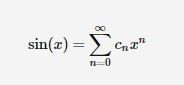# Mathjax makes creating formulae online easy

Author:  Simon Hawkesworth

Adding mathematical formulae and notation to documents and applications can be problematic as these often struggle to render the notation in the correct way. Mathjax is an online application that allows mathematical formula to be written using Latex coding. It means that you can present correct mathematical formulae and notation to your users in web browsers, web pages and VLEs.

In Blackboard VLE you can add a simple piece of Javascript code to make this work inside your online Module spaces. Once the code is added to a Blackboard Assignment, Blog or other element, using the HTML editor, you can then add mathematical notation using the Latex coding. The end result is that your users see the mathematical notation as you intended.

How to add the javascript code:

1. Create a Blackboard Assignment, Blog or other element and give it a title,
2. In the text area select the HTML editor option and paste in this code: <script src=”https://cdn.mathjax.org/mathjax/latest/MathJax.js?config=TeX-AMS-MML_HTMLorMML” type=”text/javascript”>/*<![CDATA[*//*]]>*/</script>
3. Close the HTML editor and return to the text editor option/view. It’s important that you’re now in the text editor, rather than the HTML editor, before you begin adding the content you want your users to view.
4. Add your mathematical formulae in Latex code, along with any instructions or details you want to give your students in normal text,

An example of Latex code is:

$\sin(x) = \sum_{n=0}^{\infty} c_n x^n$

When you finish and Save/Submit, the Mathjax Javascript you added earlier then renders the Latex code as mathematical formulae:Useful resources:

To find out more about using Latex coding, here are some useful websites:

Latex for beginners